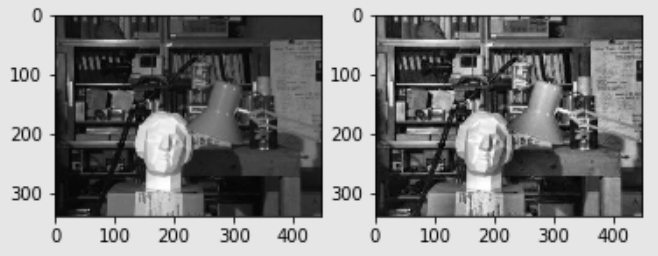WHCSRL 技术网

# opencv22:Histograms直方图均衡_uncle

• 学习直方图均衡化的概念
• 利用它来提高图像的对比度

### 理论### Numpy中的均衡化实现

``````img = cv2.imread('wiki.png', 0)
hist, bins = np.histogram(img.ravel(), 256, [0,256])
cdf = hist.cumsum()
cdf_normalized = cdf * float(hist.max()-hist.min())/cdf.max()
plt.subplot(121)
plt.imshow(img, cmap='gray')
plt.subplot(122)
plt.plot(cdf_normalized, color='b')
plt.hist(img.ravel(), 256, [0, 256], color='r')
plt.xlim([0, 256])
plt.legend(('cdf', 'historgram'), loc='upper left')
plt.show()
```123456789101112```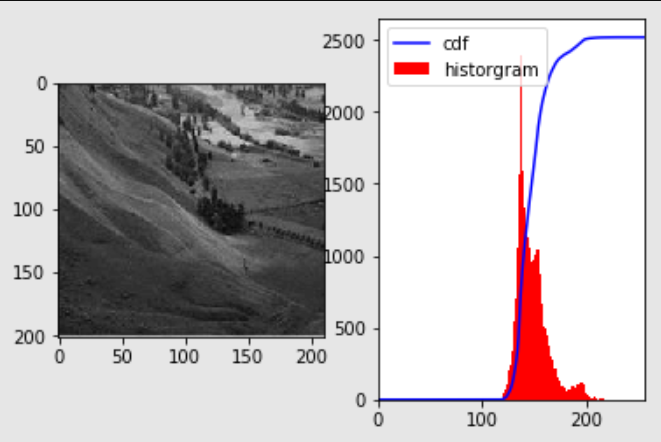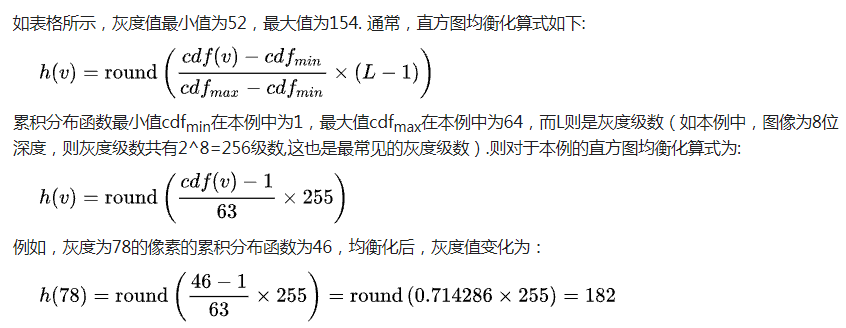``````cdf_m = np.ma.masked_equal(cdf,0)
cdf_m = (cdf_m - cdf_m.min())*255/(cdf_m.max()-cdf_m.min())
cdf = np.ma.filled(cdf_m,0).astype('uint8')
```123```

``````img2 = cdf[img]
hist, bins = np.histogram(img2.ravel(), 256, [0,256])

plt.subplot(121)
plt.imshow(img2, cmap='gray')
plt.subplot(122)
plt.plot(cdf_normalized, color='b')
plt.hist(img2.ravel(), 256, [0, 256], color='r')
plt.xlim([0, 256])
plt.legend(('cdf', 'historgram'), loc='upper left')
plt.show()
```1234567891011```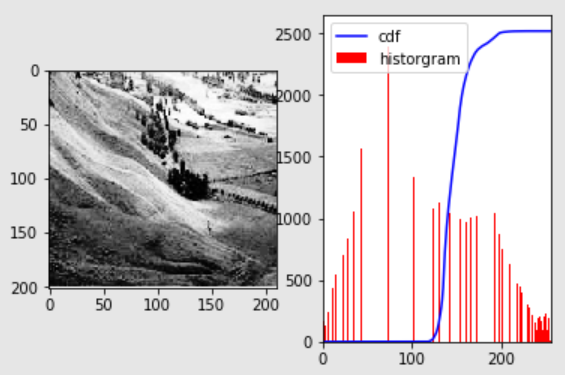### OpenCV中的直方图均衡实现

OpenCV具有执行此操作的函数`cv.equalizeHist()`。它的输入是灰度图像，输出的是直方图均衡图像。 代码如下：

``````img = cv2.imread('wiki.png', 0)
equ = cv2.equalizeHist(img)

# res = np.hstack((img, equ))  # stacking images side-by-side
plt.subplot(121)
plt.imshow(img, cmap='gray')

plt.subplot(122)
plt.imshow(equ, cmap='gray')
plt.show()
```12345678910```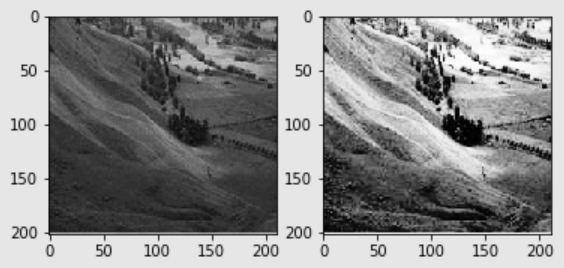### CLAHE（对比度受限的自适应直方图均衡）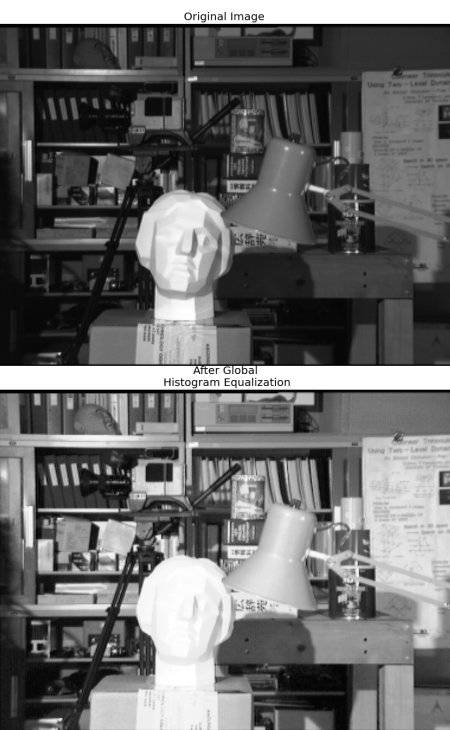``````# clahe
import cv2
import numpy as np
from matplotlib import pyplot as plt

# create a clahe object
clahe = cv2.createCLAHE(clipLimit=2.0,  tileGridSize=(8,8))
cl1 = clahe.apply(img)

plt.subplot(121)
plt.imshow(img, cmap='gray')

plt.subplot(122)
plt.imshow(cl1, cmap='gray')
plt.show()
```1234567891011121314151617```